# Conservation laws

A conservation law is a type of PDE that describes the transport of extensive quantities like mass, momentum, and energy. The most general form of a hyperbolic conservation law for a field $q$ is

$$\frac{\partial q}{\partial t} + \nabla\cdot f(q) = s,$$

where $f$ is the flux function and $s$ the sources. The solution variable $q$ could be a scalar, vector, or tensor field. Here we'll look at the simplest conservation law of them all, the advection equation: $q$ is a scalar field and $f(q) = qu$ for some velocity field $u$. As we'll see, there are lots of ways to screw up something as simple as the advection equation, so learning what the common error modes are will help when attacking harder problems like the shallow water or Euler equations.

One of the challenging things about solving hyperbolic problems is that the class of reasonable solutions includes functions with jump discontinuities, and for some problems this is true even when the initial data are smooth. Compare that to, say, elliptic problems, where the solution is almost always smoother than the input data. For elliptic problems, it's common to use continuous basis functions, but when we try to use the same basis for conservation laws we can run up against some very nasty stability problems. It's possible to work around these issues by using tricks like the streamlined upwind Petrov-Galerkin method. But almost any approach to stabilizing a CG discretization introduces (1) free parameters that can be difficult to tune right and (2) an unrealistic level of numerical diffusion. For these reasons, the discontinuous Galerkin method is very popular for these kinds of problems. The DG method has good local conservation properties, it can achieve high-order accuracy where the solution is smooth, and there are more options in how you guarantee a stable scheme.

DG is a huge subject and I couldn't possibly do justice to it here. If you want to read more about it, this paper by Cockburn and Shu is a great reference, as are these notes by Ralf Hartmann and this dissertation by Michael Crabb. Instead, I'll focus here on the effects of some of the choices you have to make when you solve these types of problems.

#### Input data¶

First, we want to create a domain, some function spaces, and a divergence-free velocity field $u$. The classic example is a material in uniform solid-body rotation around some fixed point $y$:

$$u(x) = \hat k \times (x - y)$$

where $\hat k$ is the unit vector in the $z$ direction.

import firedrake
from firedrake import inner, Constant, as_vector
mesh = firedrake.UnitSquareMesh(64, 64, diagonal='crossed')
x = firedrake.SpatialCoordinate(mesh)
y = Constant((.5, .5))
r = x - y
u = as_vector((-r, r))


To have a stable timestepping scheme, we'll need to satisfy the Courant-Friedrichs-Lewy condition, which means calculating the maximum speed and the minimum cell diameter. Calculating the maximum speed exactly can be challenging; if $u$ is represented with piecewise linear basis functions, then $|u|^2$ is a quadratic function and thus might not attain its maximum value at the interpolation points. You could work around this by changing to a basis of Bernstein polynomials, but for our purposes it'll be enough to evaluate the maximum at the interpolation points and take a smaller timestep than necessary.

import numpy as np
Q = firedrake.FunctionSpace(mesh, family='CG', degree=2)
speed = firedrake.interpolate(inner(u, u), Q)
max_speed = np.sqrt(speed.dat.data_ro.max())

Q0 = firedrake.FunctionSpace(mesh, family='DG', degree=0)
diameters = firedrake.project(firedrake.CellDiameter(mesh), Q0)
min_diameter = diameters.dat.data_ro.min()

cfl_timestep = min_diameter / max_speed
print('Upper bound for CFL-stable timestep: {}'.format(cfl_timestep))

Upper bound for CFL-stable timestep: 0.022097086912079608


The initial data we'll use will be the classic bell and cone:

$$q_0 = \max\{0, 1 - |x - x_c| / r_c\} + \max\{0, 1 - |x - x_b|^2 / r_b^2\}$$

where $x_c$, $r_c$ are the center and radius of the cone and $x_b$, $r_b$ for the bell.

from firedrake import sqrt, min_value, max_value

x_c = as_vector((5/8, 5/8))
R_c = Constant(1/8)

x_b = as_vector((3/8, 3/8))
R_b = Constant(1/8)

q_expr = (
max_value(0, 1 - sqrt(inner(x - x_c, x - x_c) / R_c**2)) +
max_value(0, 1 - inner(x - x_b, x - x_b) / R_b**2)
)
q0 = firedrake.project(q_expr, Q0)

import matplotlib.pyplot as plt
import mpl_toolkits.mplot3d
fig = plt.figure()
firedrake.trisurf(q0, axes=axes);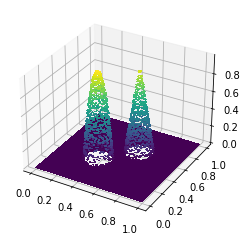#### Fluxes¶

For our first experiment we'll look at the problem of choosing a numerical flux. As we'll see in the following, we have choices to make in how we determine the discrete approximation to the solution of the conservation law. This is very different from elliptic problems -- once we've decided to use the continous Galerkin method, the only real choice is what polynomial degree we'll use.

The usual procedure to come up with a weak form for a PDE is to multiply by some smooth test function $\phi$, move some derivatives onto $\phi$, and integrate. For the conservation law written above, we would arrive at the weak form

$$\int_\Omega\left(\frac{\partial q}{\partial t}\cdot\phi - f(q)\cdot\nabla\phi\right)dx = \int_\Omega s\cdot\phi\, dx + \ldots$$

where I've used an ellipsis to stand for some boundary terms that don't particularly matter. Unfortunately, this equation doesn't quite tell the whole story. We're using discontinuous basis functions to represent the solution $q$, and ideally we would use the same basis and test functions. What happens when the test functions are discontinuous too?

Let $\phi$ be some basis function and let $K$ be the cell of the domain where $\phi$ is supported. If we apply the usual procedure, we get an element-wise weak form when integrating against $\phi$:

$$\int_K\left(\frac{\partial q}{\partial t}\phi - f(q)\cdot\nabla\phi\right)dx + \int_{\partial K}f(q)\cdot\phi n\, ds = \int_K s\cdot\phi\, dx + \ldots$$

where $n$ is the unit outward normal vector to $K$. Note that we're integrating over only a single element and not the entire domain. The problem here is that if the solution and the basis functions are discontinuous across the element, we can't uniquely define their values on the boundary.

To see why this is so, you can imagine that, instead of having a discontinuous test function, we have a sequence $\phi_\epsilon$ of continuous test functions that converge to $\phi$ in some appropriate norm. If we take the support of each element of the sequence to be contained in the interior of $K$, then the value of $q$ in the boundary integral will its the value approaching the boundary from inside:

$$q_-(x) = \lim_{\epsilon\to 0}q(x - \epsilon n).$$

Alternatively, if we take $K$ to be contained in the interior of the support of each element of the sequence, then the value of the solution in the boundary integral will be its value approach the boundary from the outside:

$$q_+(x) = \lim_{\epsilon\to 0}q(x + \epsilon n).$$

Finally, with the right choice of sequence we could get any weighted average of the values on either side of the interface. As a consequence, we need to make some choice of the numerical flux. The numerical flux $f^*$ is a function of the interface values $q_+$ and $q_-$ and the unit normal vector $n$. The discrete approximation to the solution will satisfy the ODE system

$$\sum_K\left\{\int_K\left(\frac{\partial q}{\partial t}\phi - f(q)\cdot\nabla\phi\right)dx + \int_{\partial K}f^*(q_-, q_+, n)\cdot\phi\, ds\right\} = \sum_K\int_K s\cdot\phi\, dx + \ldots$$

for all test functions $\phi$. What kinds of functions can make a good numerical flux? First, if the solution is continuous across an element boundary, the numerical flux should give the same value as the true physical flux:

$$f^*(q, q, n) = f(q)\cdot n.$$

This condition is called consistency and it guarantees that the exact solution is also a discrete solution. The second property we want is to have some analogue of the conservative nature of the true problem. The important thing about fluxes in physical problems is that they can't create or destroy mass, momentum, energy, etc., they only transport it around the domain. To see how we can attain a similar property for our discrete problem, first observe that the sum over all the boundary integrals is telescoping because two neighboring cells $K_-$, $K_+$ share a common face $E$. We can then rewrite the sum of all the boundary integrals as a sum over all faces $E$ of the mesh:

$$\sum_K\int_{\partial K}f^*(q_-, q_+, n)\phi\, ds = \sum_E\int_E\left\{f^*(q_-, q_+, n_-)\phi_- + f^*(q_+, q_-, n_+)\phi_+\right\}ds$$

here $n_-$, $n_+$ are the unit outwardn ormal vectors to $K_-$ and $K_+$ respectively. Note that $n_+ = -n_-$, i.e. the two normals point in opposite directions to each other. What happens if the test function $\phi$ is identically equal to 1 throughout the entire domain? In that case the facet integrals should sum up to 0 -- fluxes only transport, they don't create or destroy. The numerical flux is conservative if

$$f^*(q_-, q_+, n) + f^*(q_+, q_-, -n) = 0.$$

The most braindead way we can come up with a sane numerical flux is to take the average of the solution values across the cell boundary:

$$f^*(q_-, q_+, n) = \frac{1}{2}(q_- + q_+)\cdot n.$$

This is called the central flux. Let's see how well it works.

from firedrake import grad, dx, ds, dS

q, ϕ = firedrake.TrialFunction(Q0), firedrake.TestFunction(Q0)
m = q * ϕ * dx

q = q0.copy(deepcopy=True)
cell_flux = -inner(grad(ϕ), q * u) * dx

n = firedrake.FacetNormal(mesh)
f = q * inner(u, n)
face_flux = (f('+') - f('-')) * (ϕ('+') - ϕ('-')) * dS

q_in = Constant(0)
influx = q_in * min_value(0, inner(u, n)) * ϕ * ds
outflux = q * max_value(0, inner(u, n)) * ϕ * ds


We'll take our timestep to be 1/4 of the formal CFL-stable timestep. We need at least a factor of 1/2 for the dimension, and probably another factor of 1/2 for triangle shape.

from numpy import pi as π
final_time = 2 * π
num_steps = 4 * int(final_time / cfl_timestep)
dt = Constant(final_time / num_steps)


Since we're repeatedly solving the same linear system, we'll create problem and solver objects so that this information can be reused from one solve to the next. The solver parameters are specially chosen for the fact that the mass matrix with discontinuous Galerkin methods is block diagonal, so a block Jacobi preconditioner with exact solvers on all the blocks is exact for the whole system.

from firedrake import LinearVariationalProblem, LinearVariationalSolver
dq_dt = -(cell_flux + face_flux + influx + outflux)

δq = firedrake.Function(Q0)
problem = LinearVariationalProblem(m, dt * dq_dt, δq)
parameters = {'ksp_type': 'preonly', 'pc_type': 'bjacobi', 'sub_pc_type': 'ilu'}
solver = LinearVariationalSolver(problem, solver_parameters=parameters)

import numpy as np
qrange = np.zeros((num_steps, 2))

import tqdm
for step in tqdm.trange(num_steps, unit='timesteps'):
solver.solve()
q += δq
qrange[step, :] = q.dat.data_ro.min(), q.dat.data_ro.max()

100%|██████████| 1136/1136 [00:05<00:00, 225.35timesteps/s]


After only 250 steps the solution is already attaining values two orders of magnitude greater than what they should, even while using a CFL-stable timestep. The reason for this is that the central flux, while both consistent and conservative, is numerically unstable with forward time-differencing.

fig, axes = plt.subplots()
axes.set_yscale('log')
axes.plot(qrange[:250, 1])
axes.set_xlabel('timestep')
axes.set_ylabel('solution maximum');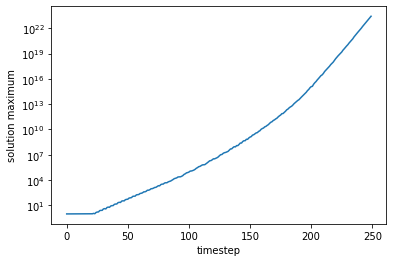Instead, we'll try the upwind numerical flux. The idea of the upwind flux is to sample from whichever side of the interface has the velocity flowing outward and not in. The numerical flux is defined as

$$f^*(q_-, q_+, n) = \begin{cases}q_-u\cdot n && u\cdot n > 0 \\ q_+u\cdot n && u\cdot n \le 0\end{cases}.$$

We can also write this in a more symmetric form as

$$f^*(q_-, q_+, n) = q_-\max\{0, u\cdot n\} + q_+\min\{0, u\cdot n\}.$$

The upwind flux is designed to mimic the stability properties of one-sided finite difference schemes for transport equations.

q = q0.copy(deepcopy=True)
cell_flux = -inner(grad(ϕ), q * u) * dx

n = firedrake.FacetNormal(mesh)
u_n = max_value(inner(u, n), 0)
f = q * u_n
face_flux = (f('+') - f('-')) * (ϕ('+') - ϕ('-')) * dS

q_in = Constant(0)
influx = q_in * min_value(0, inner(u, n)) * ϕ * ds
outflux = q * max_value(0, inner(u, n)) * ϕ * ds

dq_dt = -(cell_flux + face_flux + influx + outflux)

δq = firedrake.Function(Q0)
problem = LinearVariationalProblem(m, dt * dq_dt, δq)
parameters = {'ksp_type': 'preonly', 'pc_type': 'bjacobi', 'sub_pc_type': 'ilu'}
solver = LinearVariationalSolver(problem, solver_parameters=parameters)

qs = []
output_freq = 5

for step in tqdm.trange(num_steps, unit='timesteps'):
solver.solve()
q += δq
if step % output_freq == 0:
qs.append(q.copy(deepcopy=True))

100%|██████████| 1136/1136 [00:05<00:00, 223.81timesteps/s]


We at least get a finite answer as a result, which is a big improvement. Keeping in mind that the original data capped out at a value of 1, the peaks have shrunk considerably, and we can also see that the sharp cone is much more rounded than before.

from firedrake.plot import FunctionPlotter
fn_plotter = FunctionPlotter(mesh, num_sample_points=1)

%%capture
fig, axes = plt.subplots()
axes.set_aspect('equal')
axes.get_xaxis().set_visible(False)
axes.get_yaxis().set_visible(False)
colors = firedrake.tripcolor(
q, num_sample_points=1, vmin=0., vmax=1., shading="gouraud", axes=axes
)

from matplotlib.animation import FuncAnimation
def animate(q):
colors.set_array(fn_plotter(q))

interval = 1e3 * output_freq * float(dt)
animation = FuncAnimation(fig, animate, frames=qs, interval=interval)

from IPython.display import HTML
HTML(animation.to_html5_video())


Despite this fact, the total volume under the surface has been conserved to within rounding error.

print(firedrake.assemble(q * dx) / firedrake.assemble(q0 * dx))

0.9999713508961685


Nonetheless, the relative error in the $L^1$ norm is quite poor.

firedrake.assemble(abs(q - q0) * dx) / firedrake.assemble(q0 * dx)

0.6651047426779894

Let's see if we can improve on that by changing the finite element basis.

#### Higher-order basis functions¶

One of the main advantages that the discontinuous Galerkin method has over the finite volume method is that achieving higher-order convergence is straightforward if the problem is nice -- you just increase the polynomial degree. (When the problem is not nice, for example if there are shockwaves, everything goes straight to hell and the finite volume method is much less finicky about stability.) Here we'll look at what happens when we go from piecewise constant basis functions to piecewise linear.

One of the first changes we have to make is that the Courant-Friedrichs-Lewy condition is more stringent for higher-order basis functions. For piecewise constant basis functions, we have that $\delta x / \delta t \ge |u|$; for degree-$p$ polynomials, we instead need that

$$\frac{\delta x}{\delta t} \ge (2p + 1)\cdot|u|.$$

One way of looking at this higher-degree CFL condition is that the introduction of more degrees of freedom makes the effective spacing between the nodes smaller than it might be in the piecewise-constant case. The multiplicative factor of $2p + 1$ accounts for the effective shrinkage in the numerical length scale. (For more, see this paper from 2013.) Once again, we'll use a timestep that's 1/4 of the formal CFL timestep to account for the spatial dimension and the mesh quality.

cfl_timestep = min_diameter / max_speed / 3
num_steps = 4 * int(final_time / cfl_timestep)
dt = Constant(final_time / num_steps)


We have to be a bit carefuly about creating the initial data. For discontinuous Galerkin discretizations, we would normally project the expression into the discrete function space. Since this is a projection in $L^2$, we might get negative values for an otherwise strictly positive expression. In this case, the positivity of the solution is vital and so instead I'm interpolating the expression for the initial data, but doing so is a little dangerous.

Q1 = firedrake.FunctionSpace(mesh, family='DG', degree=1)
q0 = firedrake.interpolate(q_expr, Q1)
q0.dat.data_ro.min(), q0.dat.data_ro.max()

(0.0, 1.0)

In almost every other respect the discretization is the same as before.

q, ϕ = firedrake.TrialFunction(Q1), firedrake.TestFunction(Q1)
m = q * ϕ * dx

q = q0.copy(deepcopy=True)
cell_flux = -inner(grad(ϕ), q * u) * dx

n = firedrake.FacetNormal(mesh)
u_n = max_value(inner(u, n), 0)
f = q * u_n
face_flux = (f('+') - f('-')) * (ϕ('+') - ϕ('-')) * dS

q_in = Constant(0)
influx = q_in * min_value(0, inner(u, n)) * ϕ * ds
outflux = q * max_value(0, inner(u, n)) * ϕ * ds

dq_dt = -(cell_flux + face_flux + influx + outflux)

δq = firedrake.Function(Q1)
problem = LinearVariationalProblem(m, dt * dq_dt, δq)
parameters = {'ksp_type': 'preonly', 'pc_type': 'bjacobi', 'sub_pc_type': 'ilu'}
solver = LinearVariationalSolver(problem, solver_parameters=parameters)

for step in tqdm.trange(num_steps, unit='timesteps'):
solver.solve()
q += δq

100%|██████████| 3412/3412 [00:40<00:00, 83.82timesteps/s]


The error in the $L^1$ norm is less than that of the degree-0 solution, which was on the order of 40%, but it's far from perfect.

firedrake.assemble(abs(q - q0) * dx) / firedrake.assemble(q0 * dx)

0.09376446683007597

Worse yet, the final value of the solution has substantial over- and undershoots. The mathematical term for this is that the true dynamics are monotonicity-preserving -- they don't create new local maxima or minima -- but the numerical scheme is not.

fig, axes = plt.subplots()
axes.set_aspect('equal')
colors = firedrake.tripcolor(q, axes=axes)
fig.colorbar(colors);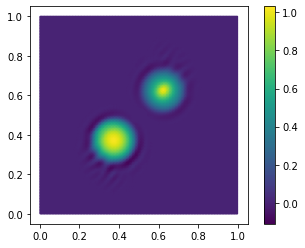To be precise and for later comparison we'll print out exactly how far outside the initial range the solution goes.

q.dat.data_ro.min(), q.dat.data_ro.max()

(-0.11039252600936499, 1.0315252284314207)

But of course we're only using the explicit Euler timestepping scheme, which is of first order, while our spatial discretization should be 2nd-order accurate. Can we do better if we match the asymptotic accuracy of the errors in time and space?

#### Timestepping¶

Choosing a finite element basis or a numerical flux is part of deciding how we'll discretize the spatial part of the differential operator. After that we have to decide how to discretize in time. The explicit Euler, which we used in the preceding code, has the virtue of simplicity. Next we'll try out the strong stability-preserving Runge-Kutta method of order 3. First, we'll create a form representing the rate of change of $q$ with the upwind flux just as we did before.

q = q0.copy(deepcopy=True)
ϕ = firedrake.TestFunction(Q1)
cell_flux = -inner(grad(ϕ), q * u) * dx

n = firedrake.FacetNormal(mesh)
u_n = max_value(inner(u, n), 0)
f = q * u_n
face_flux = (f('+') - f('-')) * (ϕ('+') - ϕ('-')) * dS

q_in = Constant(0)
influx = q_in * min_value(0, inner(u, n)) * ϕ * ds
outflux = q * max_value(0, inner(u, n)) * ϕ * ds

dq_dt = -(cell_flux + face_flux + influx + outflux)


To implement the SSPRK3 timestepping scheme, we'll introduce some auxiliary functions and solvers for the Runge-Kutta stages.

q1 = firedrake.Function(Q1)
q2 = firedrake.Function(Q1)

F2 = firedrake.replace(dq_dt, {q: q1})
F3 = firedrake.replace(dq_dt, {q: q2})

problems = [
LinearVariationalProblem(m, dt * dq_dt, δq),
LinearVariationalProblem(m, dt * F2, δq),
LinearVariationalProblem(m, dt * F3, δq)
]

solvers = [
LinearVariationalSolver(problem, solver_parameters=parameters)
for problem in problems
]


The timestepping loop is more involved; we have to separately evaluate the Runge-Kutta stages and then form the solution as an appropriate weighted sum.

for step in tqdm.trange(num_steps, unit='timesteps'):
solvers.solve()
q1.assign(q + δq)

solvers.solve()
q2.assign(3 * q / 4 + (q1 + δq) / 4)

solvers.solve()
q.assign(q / 3 + 2 * (q2 + δq) / 3)

100%|██████████| 3412/3412 [02:07<00:00, 26.84timesteps/s]


The SSPRK3 scheme gives a huge improvement in how well it agrees with the true solution.

firedrake.assemble(abs(q - q0) * dx) / firedrake.assemble(q0 * dx)

0.028571053235589616

In the eyeball norm, it looks like it stays pretty well wtihin the upper and lower limits of the initial data.

fig, axes = plt.subplots()
axes.set_aspect('equal')
colors = firedrake.tripcolor(q, axes=axes)
fig.colorbar(colors);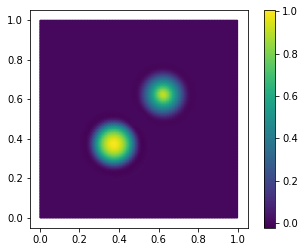But if we explicitly calculate the upper and lower bounds, we see that this scheme also fails to be monotonicity preserving!

q.dat.data_ro.min(), q.dat.data_ro.max()

(-0.023255380690921732, 1.0038686288761318)

The departures are relatively small but for more challenging or nonlinear problems the overshoots can become more severe. There is (unfortunately) a can't-do theorem that tells us why: the Godunov barrier. This theorem states that any linear, monotonicity-preserving scheme for hyperbolic conservation laws can be at most 1st-order accurate.

In principle this might sound like a bit of a bummer; why bother looking for higher-order accurate numerical schemes if they're doomed to do unphysical things that will likely result in instability? The operative word here is a linear scheme. The Godunov barrier does not rule out the possibility of nonlinear monotonicity-preserving schemes. I find it profoundly disturbing that we should be using nonlinear schemes to approximate the solutions of linear conservation laws, but ours is but to do and die I suppose.

#### Flux limiters¶

The Godunov barrier motivated the development in the early 80s of post-processing techniques that would turn an otherwise oscillatory scheme into one that does not introduce new local maxima or minima. These ideas fall under the aegis of flux limiters or slope limiters, which apply a transformation that clamps the solution in such a way as to suppress unrealistic gradients near sharp discontinuities but which leave the solution unaltered where it is smooth. The design of limiters is part science and part art. Sweby (1984) established some constraints on the what a good limiter function can look like in order to guarantee that the numerical scheme is variation-diminishing. But there's a very large range within those constraints; Sweby's paper showed three different ones even in 1984 and the wiki article on flux limiters lists 15.

Flux-corrected transport is a huge subject, and rather than try to do it any kind of justice I'll instead refer you to a wonderful book by Dmitri Kuzmin. Instead, let's finish things off by looking at what happens when we add a flux limiter to our simulation above. The application of the limiter will be interleaved with all of the Runge-Kutta stages, and conveniently we can reuse the existing solvers for the SSPRK3 stages.

q.assign(q0)
limiter = firedrake.VertexBasedLimiter(Q1)

for step in tqdm.trange(num_steps, unit='timesteps'):
solvers.solve()
q1.assign(q + δq)
limiter.apply(q1)

solvers.solve()
q2.assign(3 * q / 4 + (q1 + δq) / 4)
limiter.apply(q2)

solvers.solve()
q.assign(q / 3 + 2 * (q2 + δq) / 3)
limiter.apply(q)

100%|██████████| 3412/3412 [02:37<00:00, 21.72timesteps/s]


The relative error in the 1-norm is just as good as before, but with the flux limiter the solution does a much better job staying within the bounds of the initial data.

firedrake.assemble(abs(q - q0) * dx) / firedrake.assemble(q0 * dx)

0.034105170730422026
q.dat.data_ro.min(), q.dat.data_ro.max()

(1.4278749839079737e-45, 0.958887212115741)

#### Conclusion¶

Hyperbolic problems are hard. There are difficult decisions to make even at the level of how to formulate the discrete problem. For this demo, we were looking at a scalar conservation laws, and the upwind flux works quite well. But for systems of conservation laws, like the shallow water equations, things become much more involved. You have to know something at an analytical level about the underlying problem -- the wave speeds. Once we've decided which discrete problem to solve, going beyond first-order accuracy is filled with even more challenges. Some issues, like getting a stable enough timestep, often require manual tuning. For the linear problem shown here, we know what the wave speeds are from the outset and we have reasonable confidence that we can pick a good timestep that will work for the entire simulation. The solutions of nonlinear conservation laws can meander to regions of state space where the wave speeds are much higher than where they started and an initially stable timestep becomes unstable. The Right Thing To Do is to use an adaptive timestepping scheme. But you now have the added implementational difficulty of tracking a higher- and lower-order solution with which to inform the adaptation strategy. Hopefully this has shown what some of the typical pitfalls are and what tools are available to remedy them.

# Stokes flow

In the last post, we looked at variational principles by studying the minimal surface equation. Much of what you learn in multivariable calculus carries over equally well to infinite-dimensional spaces and we were able to leverage a lot of this intuition to design efficient solution procedures. For example, the notion of convexity carries over to variatonal problems and using this idea we can show that Newton's method is effective in this setting as well.

When we solved the minimal surface equation, our solution space consists of all functions that satisfy a set of Dirichlet boundary conditions. These conditions are easy to eliminate so our problem is essentially unconstrained. In this post, we'll look at the Stokes equations, which are a constrained optimization problem. For unconstrained problems, the convexity of the objective implies a kind of stability property that we can use to prove that roughly any finite element basis will give a convergent approximation scheme. For constrained problems we have to be much more careful about the choice of basis and this is the content of the Ladyzhenskaya-Babuška-Brezzi or LBB conditions, which I'll describe in a later post. For now, we'll focus on solving the Stokes equations using one particular discretization, the Taylor-Hood element.

The Stokes equations describe slow flow of very viscous, incompressible fluids. The fields we're solving for are the velocity $u$ and the pressure $p$. The incompressibility condition means that the velocity field is divergence-free:

$$\nabla\cdot u = 0.$$

The remaining equations state that the stresses are in balance wth body forces:

$$\nabla\cdot \tau - \nabla p + f = 0,$$

where $\tau$ is the rank-2 stress tensor and $f$ are the body forces. The stress tensor must be related somehow to the velocity field. For a viscous fluid, the stress tensor is related to the rate-of-strain tensor

$$\dot\varepsilon = \frac{1}{2}\left(\nabla u + \nabla u^*\right).$$

(For solids the stress tensor is related to the gradient of the displacement rather than the velocity.) The simplest constitutive relation is that of a Newtonian fluid:

$$\tau = 2\mu\dot\varepsilon,$$

where $\mu$ is the viscosity. There are other nonlinear constitutive relations, but for now we'll just consider Newtonian fluids. If $U$ and $L$ are characteristic velocity and length scales for the particular flow at hand and $\rho$ is the fluid density, the Stokes equations are a good description when the Reynolds number is much less than 1:

$$\text{Re} \equiv \frac{\rho UL}{\mu} \ll 1.$$

When the Reynolds number is closer to or larger than 1, we need to use the full Navier-Stokes equations, which includes inertial effects as well.

The Stokes equations, like the Poisson equation, have a minimization principle, but for two fields instead of one. The variational principle is that the solution $u$, $p$ is a critical point of the rate of decrease of the Gibbs free energy:

$$\dot{\mathscr{G}}(u, p) = \int_\Omega\left(\mu|\dot\varepsilon(u)|^2 - p\nabla\cdot u - f\cdot u\right)dx.$$

You can show using the usual tricks that the Euler-Lagrange equations for this functional are the Stokes equations. The free energy dissipation functional consists of a positive, quadratic term in the velocity, but the pressure $p$ only acts like a Lagrange multiplier enforcing the incompressibility condition. The lack of any positivity in the pressure is part of what makes the Stokes equations so challenging to discretize and solve. While the second derivative of the objective is still symmetric, it is no longer positive-definite.

#### Demonstration¶

Here we'll work on a classic problem of flow driven by a moving boundary. The domain will consist of a circle with two holes removed. We'll imagine that these holes are cylindrical turbines that are rotating with some fixed speed and dragging the fluid along with them. As we'll see, getting a linear solver to converge for this problem is much more challenging than for the Poisson equation.

import numpy as np
from numpy import pi as π
def add_ellipse(geometry, x, y, a, b, N, lcar):
θs = np.array([2 * π * n / N for n in range(N)])
xs, ys = x + a * np.cos(θs), y + b * np.sin(θs)
points = [geometry.add_point([x, y, 0], lcar=lcar) for x, y in zip(xs, ys)]
lines = [geometry.add_line(points[n], points[(n + 1) % N])
for n in range(N)]

return line_loop

import pygmsh
geometry = pygmsh.built_in.Geometry()

outer_line_loop = add_ellipse(geometry, x=0, y=0, a=1, b=1, N=128, lcar=1/4)
inner_loops = [
add_ellipse(geometry, x=0, y=+1/2, a=1/8, b=1/8, N=64, lcar=1/4),
add_ellipse(geometry, x=0, y=-1/2, a=1/8, b=1/8, N=64, lcar=1/4)
]

with open('mixer.geo', 'w') as geo_file:
geo_file.write(geometry.get_code())

!gmsh -2 -format msh2 -v 0 -o mixer.msh mixer.geo

import firedrake
mesh = firedrake.Mesh('mixer.msh')

import matplotlib.pyplot as plt
fig, axes = plt.subplots()
axes.set_aspect('equal')
firedrake.triplot(mesh, axes=axes)
axes.legend();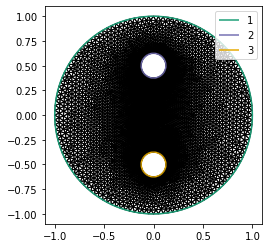For this problem we'll use the Taylor-Hood element: piecewise linear basis functions for the pressure and piecewise quadratic basis functions for the velocity. The Taylor-Hood element is stable for the Stokes equations in that norm of the inverse of the disretized linear system is bounded as the mesh is refined. This is a very special property and not just any element will work.

For scalar problems, the solution is a single field, but for the Stokes equations our solution consists of a pair of a velocity and a pressure field. Firedrake includes a handy algebraic notation for defining the direct product of two function spaces.

Q = firedrake.FunctionSpace(mesh, family='CG', degree=1)
V = firedrake.VectorFunctionSpace(mesh, family='CG', degree=2)
Z = V * Q


We can access the components of a function that lives in this product space using the usual Python indexing operators, but it's more convenient to use the function firedrake.split to give us handles for the two components.

z = firedrake.Function(Z)
u, p = firedrake.split(z)


This way our code to define the objective functional looks as much like the math as possible, rather than have to constantly reference the components.

We'll use a viscosity coefficient $\mu$ of 1000. Since the diameter of the domain and the fluid velocity are both on the order of 1, the viscosity would need to be fairly large for the Stokes equations to actually be applicable.

from firedrake import inner, sym, grad, div, dx
def ε(u):

μ = firedrake.Constant(1e3)
𝒢̇ = (μ * inner(ε(u), ε(u)) - p * div(u)) * dx


One of the extra challenging factors about the Stokes equations is that they have a non-trivial null space. To see this, suppose we have some velocity-pressure pair $u$, $p$. The velocity field $u$ is not necessarily divergence-free, but we do need that $u\cdot n = 0$ on the boundary of the domain. If we add a constant factor $p_0$ to the pressure, then the value of the objective functional is unchanged:

\begin{align} \dot{\mathscr{G}}(u, p) - \dot{\mathscr{G}}(u, p + p_0) & = \int_\Omega p_0\nabla\cdot u\, dx \\ & = p_0\int_{\partial\Omega}u\cdot n\, ds = 0. \end{align}

In order to obtain a unique solution to the system, we can impose the additional constraint that

$$\int_\Omega p\, dx = 0,$$

or in other words that the pressure must be orthogonal to all the constant functions.

from firedrake import MixedVectorSpaceBasis, VectorSpaceBasis
basis = VectorSpaceBasis(constant=True)
nullspace = MixedVectorSpaceBasis(Z, [Z.sub(0), basis])


Next we have to create the boundary conditions. The only extra work we have to do here is to get the right component of the mixed function space $Z$.

x = firedrake.SpatialCoordinate(mesh)

x2 = firedrake.as_vector((0, +1/2))
r2 = firedrake.Constant(1/8)
x3 = firedrake.as_vector((0, -1/2))
r3 = firedrake.Constant(1/8)

q2 = (x - x2) / r2
q3 = (x - x3) / r3

u2 = firedrake.as_vector((-q2, q2))
u3 = firedrake.as_vector((-q3, q3))

from firedrake import DirichletBC, as_vector
bc1 = DirichletBC(Z.sub(0), as_vector((0, 0)), 1)
bc2 = DirichletBC(Z.sub(0), u2, 2)
bc3 = DirichletBC(Z.sub(0), u3, 3)


Now let's see what happens if we invoke the default linear solver.

from firedrake import derivative

try:
firedrake.solve(
derivative(𝒢̇, z) == 0, z,
bcs=[bc1, bc2, bc3],
nullspace=nullspace
)
except firedrake.ConvergenceError:
print("Oh heavens, it didn't converge!")


We'll take the easy way out and use the sparse direct solver MUMPS to make sure we get an answer. This approach will work for now, but even parallel direct solvers scale poorly to large problems, especially in 3D. The proper incanctation to invoke the direct solver needs a bit of explaining. For mixed problems like the Stokes equations, Firedrake will assemble a special matrix type that exploits the problem's block structure. Unfortunately MUMPS can't work with this matrix format, so we have to specify that it will use PETSc's aij matrix format with the option 'mat_type': 'aij'. Next, we'll request that the solver use the LU factorization with 'pc_type': 'lu'. Without any other options, this will use PETSc's built-in matrix factorization routines. These are fine for strictly positive matrices, but fail when the problem has a non-trivial null space. The option 'pc_factor_mat_solver_type': 'mumps' will use the MUMPS package instead of PETSc's built-in sparse direct solver.

firedrake.solve(
derivative(𝒢̇, z) == 0, z,
bcs=[bc1, bc2, bc3],
nullspace=nullspace,
solver_parameters={
'mat_type': 'aij',
'ksp_type': 'preonly',
'pc_type': 'lu',
'pc_factor_mat_solver_type': 'mumps'
}
)


Some cool features you can observe in the stream plot are the saddle point at the center of the domain and the two counter-rotating vortices that form on either side of it.

u, p = z.split()
fig, axes = plt.subplots()
axes.set_aspect('equal')
kwargs = {'resolution': 1/30, 'seed': 4, 'cmap': 'winter'}
streamlines = firedrake.streamplot(u, axes=axes, **kwargs)
fig.colorbar(streamlines);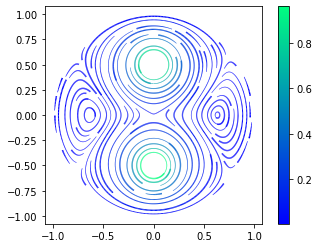The velocity field should be close to divergence-free; if we project the divergence into a DG(2) we can see what the exact value is. There are some small deviations, especially around the boundary of the domain. Part of the problem is that the boundary conditions we've specified are exactly tangent to the idealized domain -- a large circle with two circular holes punched out of it -- but not to its discrete approximation by a collection of straight edges.

S = firedrake.FunctionSpace(mesh, family='DG', degree=2)
div_u = firedrake.project(div(u), S)

fig, axes = plt.subplots()
axes.set_aspect('equal')
kwargs = {'vmin': -0.01, 'vmax': +0.01, 'cmap': 'seismic'}
triangles = firedrake.tripcolor(div_u, axes=axes, **kwargs)
fig.colorbar(triangles);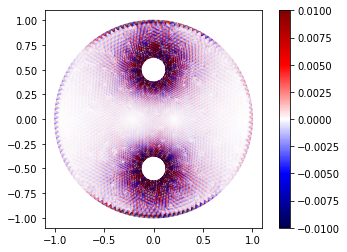For now we'll calculate and store the norm of the velocity diverence. When we try to improve on this we'll use this value as a baseline.

linear_coords_divergence = firedrake.norm(div_u)
print(linear_coords_divergence)

0.026532615307004404


#### Higher-order geometries¶

We can try to improve on this by using curved edges for the geometry instead of straight ones. The topology of the mesh is the same; we're just adding more data describing how it's embedded into Euclidean space. In principle, gmsh can generate this for us, but reading in the file seems to be awfully annoying. To get a higher-order geometry, we'll proceed by:

1. making a quadratic vector function space
2. interpolating the linear coordinates into this space
3. patching the new coordinate field to conform to the boundary

This approach will work for our specific problem but it requires us to know things about the idealized geometry that aren't always available. So what we're about to do isn't exactly generalizable.

To do the patching in step 3, we'll create boundary condition objects defined on the quadratic function space and then apply them. We need to know the numbering of the various boundary segments in order to do that, so to refresh the memory let's look at the mesh again.

fig, axes = plt.subplots()
axes.set_aspect('equal')
firedrake.triplot(mesh, axes=axes)
axes.legend();The outer curve is boundary 1, the upper mixer head is boundary 2, and the lower head is boundary 3. With that in mind we can create the new coordinate field.

Vc = firedrake.VectorFunctionSpace(mesh, family='CG', degree=2)

from firedrake import sqrt, Constant
distance = sqrt(inner(x - center, x - center))
return center + radius * (x - center) / distance

centers = [Constant((0., 0.)), Constant((0., +.5)), Constant((0., -0.5))]
bcs = [firedrake.DirichletBC(Vc, fixup(x, center, radius), index + 1)

X0 = firedrake.interpolate(mesh.coordinates, Vc)
X = X0.copy(deepcopy=True)
for bc in bcs:
bc.apply(X)


Just as a sanity check, we'll calculate the average deviation of the new from the old coordinate field to see how different they are.

from firedrake import ds
length = firedrake.assemble(Constant(1.) * ds(mesh))
firedrake.assemble(sqrt(inner(X - X0, X - X0)) * ds) / length

0.000180710597501049

Now we can solve the Stokes equations again on this new mesh using the exact same procedures as before.

qmesh = firedrake.Mesh(X)
Q = firedrake.FunctionSpace(qmesh, family='CG', degree=1)
V = firedrake.VectorFunctionSpace(qmesh, family='CG', degree=2)
Z = V * Q

z = firedrake.Function(Z)
u, p = firedrake.split(z)

𝒢̇ = (μ * inner(ε(u), ε(u)) - p * div(u)) * dx

basis = VectorSpaceBasis(constant=True)
nullspace = MixedVectorSpaceBasis(Z, [Z.sub(0), basis])

x = firedrake.SpatialCoordinate(qmesh)

x2 = firedrake.as_vector((0, +1/2))
r2 = firedrake.Constant(1/8)
x3 = firedrake.as_vector((0, -1/2))
r3 = firedrake.Constant(1/8)

q2 = (x - x2) / r2
q3 = (x - x3) / r3

u2 = firedrake.as_vector((-q2, q2))
u3 = firedrake.as_vector((-q3, q3))

from firedrake import DirichletBC, as_vector
bc1 = DirichletBC(Z.sub(0), as_vector((0, 0)), 1)
bc2 = DirichletBC(Z.sub(0), u2, 2)
bc3 = DirichletBC(Z.sub(0), u3, 3)

firedrake.solve(
derivative(𝒢̇, z) == 0, z,
bcs=[bc1, bc2, bc3],
nullspace=nullspace,
solver_parameters={
'mat_type': 'aij',
'ksp_type': 'preonly',
'pc_type': 'lu',
'pc_factor_mat_solver_type': 'mumps'
}
)

S = firedrake.FunctionSpace(qmesh, family='DG', degree=2)
div_u = firedrake.project(div(u), S)


The ring of spurious divergences around the outer edge of the domain is substantially reduced with curved elements. Nonetheless, the boundary doesn't perfectly fit the circle and this imperfection means that at some points around the edge the discretized velocity field will have an unphysical, non-zero normal component.

fig, axes = plt.subplots()
axes.set_aspect('equal')
triangles = firedrake.tripcolor(div_u, axes=axes, **kwargs)
fig.colorbar(triangles);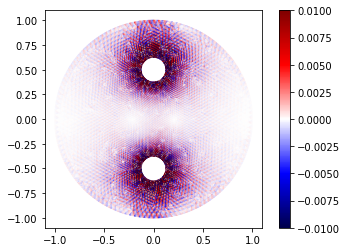Using a higher-order geometry reduced the norm of the velocity divergence almost by a factor of 4, which is a big improvement.

quadratic_coords_divergence = firedrake.norm(div_u)

3.6951400937053247


#### Conclusion¶

The code above shows how to get an exact (up to rounding-error) solution to the discretized Stokes equations using MUMPS. For larger problems in 3D, using a direct method can become prohibitively expensive. The Firedrake documentation has a demo of how to use PETSc's field split preconditioners, together with matrix-free operators, to solve the Stokes equations efficiently. In subsequent posts, I'll show more about stable discretizations of mixed problems, and how to solve the Stokes equations with more exotic boundary conditions than the standard ones we've shown here.

The velocity field we calculated was not exactly divergence-free and part of this was a consequence of using a boundary condition that adapted poorly to a piecewise-linear discretized geometry. We were able to do better by increasing the polynomial degree of the geometry, and in general this is absolutely necessary to achieve the expected rates of convergence with higher-order finite element bases. Nonetheless, the support for higher-order geometries in common finite element and mesh generation packages should be better given how useful they are. I think this is an area where a little investment in resources could make a really outsized difference. The logical endpoint of this line of thinking is isogeometric analysis, which is an active area of research.

# The obstacle problem

In this post, we'll look at the obstacle problem. We've seen in previous posts examples of variational problems -- minimization of some functional with respect to a field. The classic example of a variational problem is to find the function $u$ that minimizes the Dirichlet energy

$$\mathscr{J}(u) = \int_\Omega\left(\frac{1}{2}|\nabla u|^2 - fu\right)dx$$

subject to the homogeneous Dirichlet boundary condition $u|_{\partial\Omega} = 0$. The Poisson equation is especially convenient because the objective is convex and quadratic. The obstacle problem is what you get when you add the additional constraint

$$u \ge g$$

throughout the domain. More generally, we can look at the problem of minimizing a convex functional $\mathscr{J}$ subject to the constraint that $u$ has to live in a closed, convex set $K$ of a function space $Q$. For a totally unconstrained problem, $K$ would just be the whole space $Q$.

Newton's method with line search is a very effective algorithm for solving unconstrained convex problems, even for infinite-dimensional problems like PDEs. Things get much harder when you include inequality constraints. To make matters worse, much of the literature you'll find on this subject is focused on finite-dimensional problems, where techniques like the active-set method work quite well. It's not so obvious how to generalize these methods to variational problems. In the following, I'll follow the approach in section 4.1 of this paper by Farrell, Croci, and Surowiec, whch was my inspiration for writing this post.

Minimizing the action functional $\mathscr{J}$ over the convex set $K$ can be rephrased as an unconstrained problem to minimize the functional

$$\mathscr{J}(u) + \mathscr{I}(u),$$

where $\mathscr{I}$ is the indicator function of the set $K$:

$$\mathscr{I}(u) = \begin{cases}0 & u \in K \\ \infty & u \notin K\end{cases}.$$

This functional is still convex, but it can take the value $\infty$. The reformulation isn't especially useful by itself, but we can approximate it using the Moreau envelope. The envelope of $\mathscr{I}$ is defined as

$$\mathscr{I}_\gamma(u) = \min_v\left(\mathscr{I}(v) + \frac{1}{2\gamma^2}\|u - v\|^2\right).$$

In the limit as $\gamma \to 0$, $\mathscr{I}_\gamma(u) \to \mathscr{I}(u)$. The Moreau envelope is much easier to work with than the original functional because it's differentiable. In some cases it can be computed analytically; for example, when $\mathscr{I}$ is an indicator function,

$$\mathscr{I}_\gamma(u) = \frac{1}{2\gamma^2}\text{dist}\,(u, K)^2$$

where $\text{dist}$ is the distance to a convex set. We can do even better for our specific case, where $K$ is the set of all functions greater than $g$. For this choice of $K$, the distance to $K$ is

$$\text{dist}(u, K)^2 = \int_\Omega(u - g)_-^2dx,$$

where $v_- = \min(v, 0)$ is the negative part of $v$. So, our approach to solving the obstacle problem will be to find the minimzers of

$$\mathscr{J}_\gamma(u) = \int_\Omega\left(\frac{1}{2}|\nabla u|^2 - fu\right)dx + \frac{1}{2\gamma^2}\int_\Omega(u - g)_-^2dx$$

as $\gamma$ goes to 0. I've written things in such a way that $\gamma$ has units of length. Rather than take $\gamma$ to 0 we can instead stop at some fraction of the finite element mesh spacing. At that point, the errors in the finite element approximation are comparable to the distance of the approximate solution to the constraint set.

This is a lot like the penalty method for optimization problems with equality constraints. One of the main practical considerations when applying this regularization method is that the solution $u$ only satisfies the inequality constraints approximately. For the obstacle problem this deficiency isn't so severe, but for other problems we may need the solution to stay strictly feasible. In those cases, another approach like the logarithmic barrier method might be more appropriate.

#### Demonstration¶

For our problem, the domain will be the unit square and the obstacle function $g$ will be the upper half of a sphere.

import firedrake
nx, ny = 64, 64
Q = firedrake.FunctionSpace(mesh, family='CG', degree=1)

from firedrake import max_value, sqrt, inner, as_vector, Constant

def make_obstacle(mesh):
x = firedrake.SpatialCoordinate(mesh)
y = as_vector((1/2, 1/2))
z = 1/4
return sqrt(max_value(z**2 - inner(x - y, x - y), 0))

g = firedrake.interpolate(make_obstacle(mesh), Q)

import matplotlib.pyplot as plt
import mpl_toolkits.mplot3d
fig = plt.figure()
firedrake.trisurf(g, axes=axes);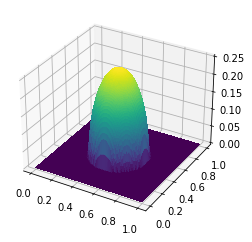Next we'll make a few utility procedures to create the Moreau envelope of the objective functional and to calculate a search direction from a given starting guess.

from firedrake import grad, dx, min_value

def make_objective(u, g, γ):
J_penalty = 0.5 / γ**2 * min_value(u - g, 0)**2 * dx
return J_elastic + J_penalty

from firedrake import derivative
def update_search_direction(J, u, v):
F = derivative(J, u)
H = derivative(F, u)

bc = firedrake.DirichletBC(u.function_space(), 0, 'on_boundary')
params = {'ksp_type': 'cg', 'pc_type': 'icc'}
firedrake.solve(H == -F, v, bc, solver_parameters=params)


Let's start from a zero initial guess and see what the first search direction will be.

u = firedrake.Function(Q)
γ = Constant(1.)
J = make_objective(u, g, γ)

v = firedrake.Function(Q)
update_search_direction(J, u, v)

fig = plt.figure()
firedrake.trisurf(v, axes=axes);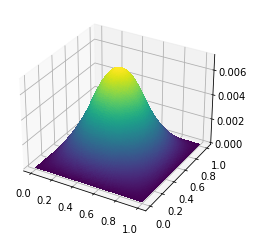To make sure that a Newton-type method will converge, we'll need a routine to perform a 1D minimization along the search direction.

from scipy.optimize import minimize_scalar
from firedrake import assemble, replace

def line_search(J, u, v):
def J_line(step):
t = firedrake.Constant(step)
J_t = replace(J, {u: u + t * v})
return assemble(J_t)

result = minimize_scalar(J_line)
assert result.success
return result.x

t = line_search(J, u, v)
print(t)

1.0000833865577647


With these steps out of the way we can define a Newton search procedure and calculate a solution for our initial, rough guess of $\gamma$.

from firedrake import action
def newton_search(J, u, tolerance=1e-10, max_num_steps=30):
v = firedrake.Function(u.function_space())
F = derivative(J, u)

for step in range(max_num_steps):
update_search_direction(J, u, v)

Δ = assemble(action(F, v))
if abs(Δ) < tolerance * assemble(J):
return

t = Constant(line_search(J, u, v))
u.assign(u + t * v)

newton_search(J, u)

fig = plt.figure()
firedrake.trisurf(u, axes=axes);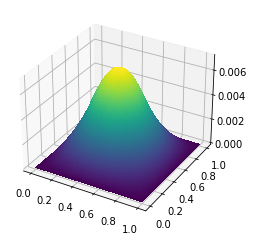The solution we obtain doesn't do a good job of staying above the obstacle because we haven't used a sufficiently small value of $\gamma$.

δ = firedrake.interpolate(max_value(g - u, 0), Q)
print(firedrake.assemble(δ * dx) / firedrake.assemble(g * dx))

0.9680039453017546


Instead, we can use the solution obtained for one value of $\gamma$ to initialize a search for the solution with $\gamma / 2$ and iterate. We've chosen this slightly indirect route rather than start from a small value of $\gamma$ directly because the problem may be very poorly conditioned. The numerical continuation approach can still give a reasonable answer even for poorly-conditioned problems.

def continuation_search(g, γ0, num_steps, contraction=0.5):
u = g.copy(deepcopy=True)
γ = Constant(γ0)

for step in range(num_steps):
J = make_objective(u, g, γ)
newton_search(J, u)
γ.assign(contraction * γ)

return u


We'll choose a number of steps so that the final value of $\gamma$ is roughly proportional to the mesh spacing.

import numpy as np
num_steps = int(np.log2(nx)) + 1
print(num_steps)

u = continuation_search(g, 1., num_steps)

7


Finally, I'll plot a cross section of the solution and the constraint $g$ so that you can see where the two coincide.

fig, axes = plt.subplots()
num_points = 51
xs = np.linspace(0., 1., num_points)
ys = 0.5 * np.ones(num_points)
X = np.array((xs, ys)).T
axes.plot(xs, g.at(X), color='tab:orange')
axes.plot(xs, u.at(X), color='tab:blue');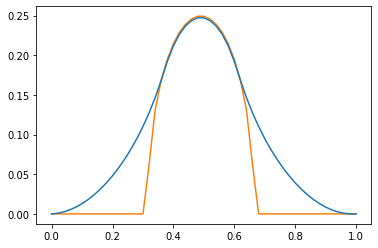#### Refinement¶

The code above worked well enough for a single grid, but one of the hard parts about optimization with PDE constraints is making sure that our algorithms do sane things under mesh refinement. Many common algorithms can have different convergence rates depending on the mesh or the degree of the finite element basis. The reasons for this are a little involved, but if you want to read more, I recommend this book by Málek and Strakos.

To really make sure we're doing things right, we should run this experiment at several levels of mesh refinement. We can do this easily using the MeshHierarchy function in Firedrake.

coarse_mesh = firedrake.UnitSquareMesh(nx, ny, quadrilateral=True)
num_levels = 3
mesh_hierarchy = firedrake.MeshHierarchy(coarse_mesh, num_levels)

for level, mesh in enumerate(mesh_hierarchy):
Q = firedrake.FunctionSpace(mesh, family='CG', degree=1)
g = firedrake.interpolate(make_obstacle(mesh), Q)
num_continuation_steps = int(np.log(nx)) + level + 1
u = continuation_search(g, 1, num_continuation_steps)
print(assemble(max_value(g - u, 0) * dx))

0.0034424020045330894
0.0009409740393031862
0.00024126075715710023
6.073519021737773e-05


If we plot the volume of the region where $u$ is less than $g$, it decreases roughly by a factor of four on every mesh refinement. This rate of decrease makes sense -- the area of each cell decreases by the same amount on each refinement. Doing a more thorough convergence study would require more computational power, but for now this is a promising sign that our algorithm works right.

#### Discussion¶

We were able to get a convergent approximation scheme for the obstacle problem by expressing the constraint as an indicator functional and then using Moreau-Yosida regularization. The idea of regularizing non-smooth optimization problems is a more general trick; we can use it for things like $L^1$ or total variation penalties as well. The Moreau envelope is another angle to look at proximal algorithms from too.

For the obstacle problem, regularization made it possible to describe every part of the algorithm using higher-level concepts (fields, functionals) without having to dive down to lower levels of abstraction (matrices, vectors). In order to implement other approaches, like the active set method, we would have no choice but to pull out the PETSc matrices and vectors that lie beneath, which is a more demanding prospect.

# Variational calculus

In this post I'll look at a classic example of a convex variational problem: computing minimal surfaces. The minimal surface problem has a simple physical interpretation in terms of soap films. Suppose you have a wire loop and you stretch a film of soap over it; what shape does the film take? The available energy that the film has to do mechanical work is proportional to the product of the surface tension and the area of the film. When the film is in equilibrium, it will minimize the energy, so it will find the surface of least area that stretches over the hoop. This shape is called a minimal surface.

Here we'll look at a geometrically simpler case where the surface can be described as the graph of a function defined on some footprint domain $\Omega$ that lives in the plane. We'll describe the position of the hoop as a function $g$ that maps the boundary $\partial\Omega$ to the reals, and the surface as a function $u$ on $\Omega$. The surface area of the graph of $u$ is the quantity

$$J(u) = \int_\Omega\sqrt{1 + |\nabla u|^2}\,dx.$$

So, our goal is to minimize the objective functional $J$ among all functions $u$ such that $u|_{\partial\Omega} = g$. This is a classic example in variational calculus, which I'll assume you're familiar with. If you haven't encountered this topic before, I learned about it from Weinstock's book.

The weak form of the Euler-Lagrange equation for $J$ is

$$\int_\Omega\frac{\nabla u\cdot\nabla v}{\sqrt{1 + |\nabla u|^2}}dx = 0$$

for all $v$ that vanish on the boundary. This PDE is just a specific way of stating the general condition that, for $u$ to be an extremum of $J$, its directional derivative along all perturbations $v$ must be 0:

$$\langle dJ(u), v\rangle = 0.$$

We can go a little bit further and calculate the second derivative of $J$ too:

$$\langle d^2J(u)\cdot v, w\rangle = \int_\Omega\frac{I - \frac{\nabla u\cdot \nabla u^*}{1 + |\nabla u|^2}}{\sqrt{1 + |\nabla u|^2}}\nabla v\cdot \nabla w\, dx,$$

Deriving this equation takes a bit of leg work, but the important part is that it looks like a symmetric, positive-definite elliptic operator, only the conductivity tensor depends on the gradient of $u$. Since the second derivative of $J$ is positive-definite, the minimization problem is convex and thus has a unique solution.

There are many approaches you could take to solving the minimal surface equation. I'll examine some here using the finite element modeling package Firedrake. If you're unfamiliar with Firedrake or FEniCS, their main selling point is that, rather than write code to fill matrices and vectors yourself, these packages use an embedded domain-specific language to describe the weak form of the PDE. The library then generates efficient C code on the spot to fill these matrices and vectors. Having done all this by hand for several years I can tell you this is a big improvement!

import firedrake


To keep things simple, we'll use the unit square as our spatial domain, and we'll use piecewise quadratic finite elements.

mesh = firedrake.UnitSquareMesh(100, 100, quadrilateral=True)
Q = firedrake.FunctionSpace(mesh, family='CG', degree=2)


I'll use a test case from some course notes from a class that Douglas Arnold teaches on finite element methods. The boundary curve is

$$g = ax\cdot\sin\left(\frac{5}{2}\pi y\right).$$

In the notes, Arnold uses $a = 1/5$. When the numerical range of $g$ is small relative to the diameter of the domain, the minimal surface equation linearizes to the Laplace equation. I want to instead look at the more nonlinear case of $a > 1$, which will stress the nonlinear solver a good deal more.

x, y = firedrake.SpatialCoordinate(mesh)

from numpy import pi as π
from firedrake import sin
a = firedrake.Constant(3/2)
g = a * x * sin(5 * π * y / 2)


A picture is worth a thousand words of course.

import matplotlib.pyplot as plt
import mpl_toolkits.mplot3d
fig = plt.figure()
firedrake.trisurf(firedrake.interpolate(g, Q), axes=axes);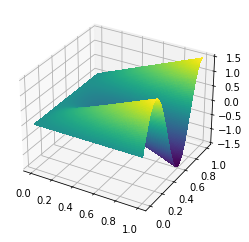Here we'll create the proposed solution $u$, define the objective functional, and try to find the minimizer naively using Firedrake's built-in solver. With the value for $a$ that I chose, the solver won't converge using its default settings.

u = firedrake.interpolate(g, Q)
bc = firedrake.DirichletBC(Q, g, 'on_boundary')

from firedrake import sqrt, inner, grad, dx
F = firedrake.derivative(J, u)

try:
firedrake.solve(F == 0, u, bc)
except firedrake.ConvergenceError:
print('Woops, nonlinear solver failed to converge!')

Woops, nonlinear solver failed to converge!


We could tweak these settings to make the solver converge, but instead let's try and dive deeper into what does and doesn't make for a good nonlinear solver.

##### Picard's method¶

This method is predicated on the idea that many nonlinear PDEs look like a linear problem with coefficients that depend on the solution. If you freeze those coefficients at the current guess for the solution, you get something that's fairly easy to solve and hopefully convergent. Suppose we've got a guess $u_n$ for the solution of the minimal surface equation. The Picard method would give us a next guess $u_{n + 1}$ that solves the linear PDE

$$\int_\Omega\frac{\nabla u_{n + 1}\cdot\nabla v}{\sqrt{1 + |\nabla u_n|^2}}dx = 0$$

for all $v$ that vanish on the boundary. This method is easy to implement if you know the functional form of the problem you're solving. Let's see how fast this decreases the area.

u.interpolate(g)
u_n = u.copy(deepcopy=True)
v = firedrake.TestFunction(Q)

import numpy as np
num_iterations = 24
Js = np.zeros(num_iterations)
Js = firedrake.assemble(J)
for step in range(1, num_iterations):
firedrake.solve(G == 0, u, bc)
u_n.assign(u)
Js[step] = firedrake.assemble(J)


The method converges in the eyeball norm in about 6 iterations.

fig, axes = plt.subplots()
axes.scatter(list(range(num_iterations)), Js, label='surface area')
axes.set_xlabel('iteration')

axes = axes.twinx()
axes.scatter(list(range(1, num_iterations)), -np.diff(Js) / Js[1:],
color='tab:orange', label='relative decrease')
axes.set_ylim(1e-6, 1)
axes.set_yscale('log')

fig.legend(loc='upper center');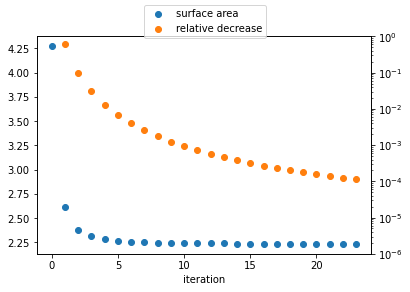This looks pretty good -- the iterates converge very rapidly to the minimizer. There are still reasons to look for something better though. Picard's method relies on the problem having special structure, which is true of the minimal surface equation but harder to find for other problems.

##### Newton's method (take 1)¶

One of the best known methods is due to Newton. The idea behind Newton's method is to use the Taylor expansion of the objective at the current guess $u_{n - 1}$ up to second order to define a quadratic approximation to the objective:

$$J(u_n + v) = J(u_n) + \langle F, v\rangle + \frac{1}{2}\langle Hv, v\rangle + \ldots$$

where $F = dJ(u_n)$, $H = d^2J(u_n)$ are the first and second derivatives of the objective. We can then define a new iterate as the minimizer of this quadratic problem:

$$u_{n + 1} = u_n + \text{argmin}_v\, \langle F, v\rangle + \frac{1}{2}\langle Hv, v\rangle.$$

The big advantage of Newton's method is that, for a starting guess sufficiently close to the solution, the iterates converge quadratically to the minimizer. Picard's method converges at best linearly.

One of the advantages of Newton's method is that there are many software packages for automatically calculating first and second derivatives of nonlinear functionals. So it's easy to apply to a broad class of problems. It isn't quite so clear how to select the right linear operator for Picard's method.

u.interpolate(g)

F = firedrake.derivative(J, u)
H = firedrake.derivative(F, u)

v = firedrake.Function(Q)

num_iterations = 24
Js = np.zeros(num_iterations + 1)
Js = firedrake.assemble(J)

bc = firedrake.DirichletBC(Q, 0, 'on_boundary')
params = {'ksp_type': 'cg', 'pc_type': 'icc'}
try:
for step in range(1, num_iterations):
firedrake.solve(H == -F, v, bc, solver_parameters=params)
u += v
Js[step] = firedrake.assemble(J)
except firedrake.ConvergenceError:
print('Newton solver failed after {} steps!'.format(step))

Newton solver failed after 3 steps!


Doesn't bode very well does it? Let's see what the objective functional did before exploding:

print(Js[:step])

[   4.27174587   13.03299587 2847.93224483]


Not a lot to save from the wreckage here -- the objective functional was increasing, which is just the opposite of what we want. What happened? Newton's method will converge quadratically if initialized close enough to the true solution. We don't have any idea a priori if we're close enough, and if we aren't then there's no guarantee that the iterates will converge at all. The example from Doug Arnold's course notes used a much smaller amplitude $a$ in the boundary data, so the initial guess is already within the convergence basin.

##### Newton's method (take 2)¶

But there's always hope! Suppose $v$ is a function such that the directional derivative of $J$ at $u$ along $v$ is negative:

$$\langle dJ(u), v\rangle < 0.$$

Then there must be some sufficiently small real number $t$ such that

$$J(u + t\cdot v) < J(u).$$

If we do have a descent direction in hand, then the problem of finding a better guess $u_{n + 1}$ starting from $u_n$ is reduced to the one-dimensional problem to minimize $J(u_n + t\cdot v)$ with respect to the real variable $t$.

If $H$ is any symmetric, positive-definite linear operator, then

$$v = -H^{-1}dJ(u)$$

is a descent direction for $J$. While the pure Newton method can diverge for some starting guesses, it does offer up a really good way to come up with descent directions for convex problems because the second derivative of the objective is positive-definite. This suggests the following algorithm:

\begin{align} v_n & = -d^2J(u_n)^{-1}dJ(u_n) \\ t_n & = \text{argmin}_t\, J(u_n + t\cdot v_n) \\ u_{n + 1} & = u_n + t_n\cdot v_n. \end{align}

This is called the damped Newton method or the Newton line search method. We can use standard packages like scipy to do the 1D minimization, as I'll show below.

u.interpolate(g)

F = firedrake.derivative(J, u)
H = firedrake.derivative(F, u)

v = firedrake.Function(Q)
bc = firedrake.DirichletBC(Q, 0, 'on_boundary')

import scipy.optimize
t = firedrake.Constant(1)
def J_t(s):
t.assign(s)
return firedrake.assemble(firedrake.replace(J, {u: u + t * v}))

num_iterations = 24
Js = np.zeros(num_iterations)
ts = np.zeros(num_iterations)
Δs = np.zeros(num_iterations)
Js = firedrake.assemble(J)

from firedrake import action
for step in range(1, num_iterations):
firedrake.solve(H == -F, v, bc, solver_parameters=params)
Δs[step] = firedrake.assemble(-action(F, v))

line_search_result = scipy.optimize.minimize_scalar(J_t)
if not line_search_result.success:
raise firedrake.ConvergenceError('Line search failed at step {}!'
.format(step))
t_min = firedrake.Constant(line_search_result.x)
u.assign(u + t_min * v)

ts[step] = t_min
Js[step] = firedrake.assemble(J)


The same convergence plot as above for Newton's method paints a very different picture.

fig, axes = plt.subplots()
axes.scatter(list(range(num_iterations)), Js, label='surface area')
axes.set_xlabel('iteration')

axes = axes.twinx()
axes.scatter(list(range(1, num_iterations)), -np.diff(Js) / Js[1:],
color='tab:orange', label='relative decrease')
axes.set_ylim(1e-16, 1)
axes.set_yscale('log')

fig.legend(loc='upper center');